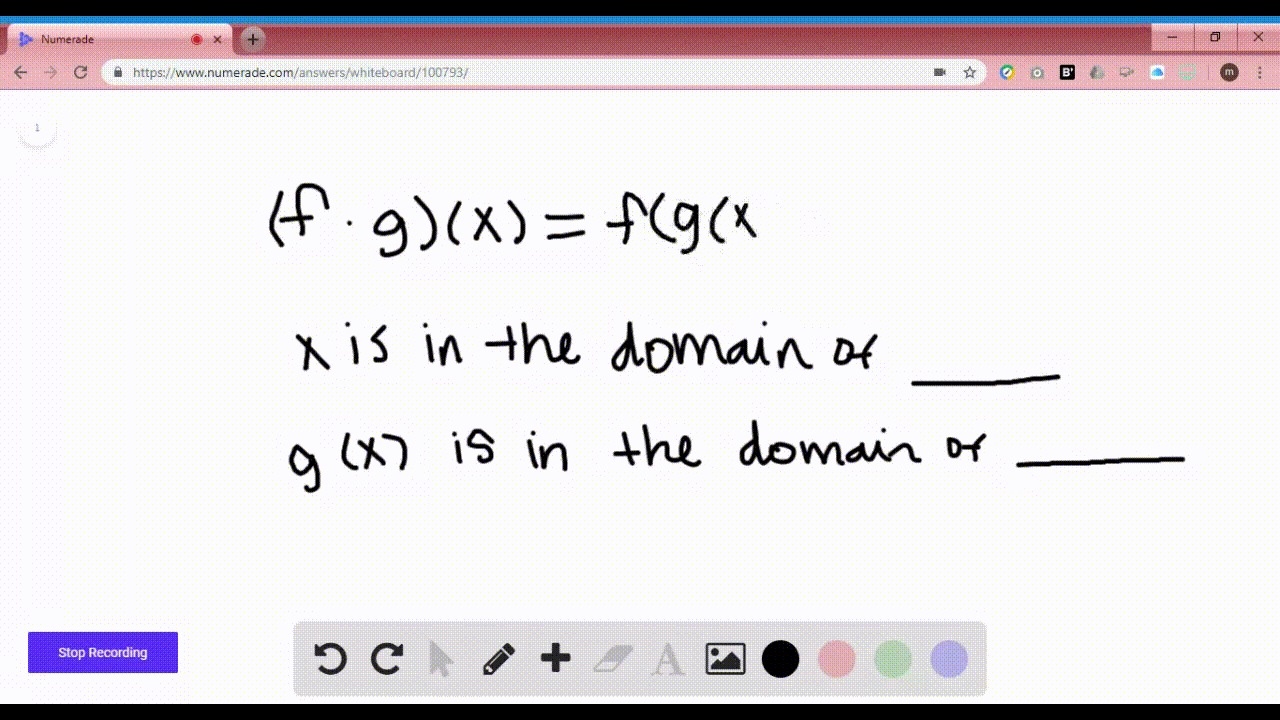Enroll in one of our FREE online STEM summer camps. Space is limited so join now!View Summer Courses### $5-6=$ Let $f$ and $g$ be functions. \begin{equa…

00:58Syracuse University

Need more help? Fill out this quick form to get professional live tutoring.

Get live tutoring
Problem 5

$5-6=$ Let $f$ and $g$ be functions.
\begin{equation}\begin{array}{l}{\text { (a) The function }(f+g)(x) \text { is defined for all values of } x} \\ {\text { that are in the domains of both } \_\_\_\_\_ \text { and }} \_\_\_\_\_. \end{array}\end{equation}
\begin{equation}\begin{array}{l}{\text { (b) The function }(f g)(x) \text { is defined for all values of } x \text { that are }} \\ {\text { in the domains of both } \_\_\_\_\_ \text { and }} \_\_\_\_\_. \end{array}\end{equation}
\begin{equation}\begin{array}{l}{\text { (c) The function }(f / g)(x) \text { is defined for all values of } x \text { that }} \\ {\text { are in the domains of both } \_\_\_\_\_ \text { and }} \_\_\_\_\_ \\ {g(x) \text { is not equal to }} \_\_\_\_\_. \end{array}\end{equation}

a) The function $(f+g)(x)$ is defined for all values of $x$ that are in the
domains of both $\underline{f}$ and $\underline{g}$
b) The function $(f g)(x)$ is defined for all values of $x$ that are in the domains
of both $f$ and $\underline{g} .$
c) The function $(f / g)(x)$ is defined for all values of $x$ that are in the domains
of both $f$ and $g,$ and $g(x)$ is not equal to $\underline{0}$ .

## Discussion

You must be signed in to discuss.

## Video Transcript

So in this problem, we're being asked to fill in the blanks. So were initially told that effin gear functions so in part A, it reads the function F plus G of X is defined for all value of values of X that are in the domains of both blank and blank. Well, when we do the addition of two functions, the domain has to be in both our domains for our two functions. So, therefore are blanks will be filled in with F and N G, because the domain would have to be in both our domain for F and G. Now, Part B is very similar. The function F times G of X is defined for all values of X that are in the domains off, both blank and blank. Well again, it has to be defined for the domains of both F and G because if it's not defined in either individual function, it won't be defined when their combined now very similar for fartsy. Except we have this extra piece. Well, it says the function F divided by G of X is defined for all values of X that are in the domains of both blank and Blank and G of X is not equal to blink well again are both of those functions. It has to be defined, so there has to be in the domains for both F and G. But G of X is not equal to what Well, because G of X would be in the denominator of our fraction G of X cannot equal to zero or else it would be undefined.